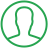# Convert List Of Int To String Python

Last Updated : Jul 1, 2023In this article we will show you the solution of convert list of int to string python, Python lists can include a wide range of object types, including integer, character, and float.

A list is an ordered series. In other programming languages, an array is the equivalent of a list, and vice versa in Python.

A comma (,) is used to denote the separation of two items in the list, and it is shown in square brackets.

The list or an array in many other programming languages differs in that a list in Python can store several data types at once, making it either homogeneous or heterogeneous, whereas an array in those other programming languages only stores a similar data type, making it homogeneous in nature.

## Step By Step Guide On Convert List Of Int To String Python :-

One variable can hold a number of objects thanks to lists. You must utilise the append() function offered by the list in order to save additional items.

Using a variety of methods, it is simple to convert an existing list containing ints to a string type in Python.

### Method - Using map()

```list_int = [1, 3, 8, 11, 13, 21, ]
list_string = map(str, list_int)
print(list(list_string))
```
1. As you can see, we use the map() method to convert a list of strings into a sorted list of integers.
2. We initialise the list with the integer data type in the first code line.
3. Next, we implement the map() function. The map() method in the Python programming language takes two arguments: one function parameter and one input value argument (list).
4. In order to turn the result into a list type, the map() method must also be wrapped in a list() call.
5. Finally, we use the print function to output a sorted list of those integers.

### Method - Using Recursive method.

```list_int = [1, 12, 15, 21, 131]
def convert_to_int(lst):
if len(lst) == 0:
return []
else:
return [str(lst)] + convert_to_int(lst[1:])
list_int_sorted = sorted(list_int)
list_int = convert_to_int(list_int_sorted)
print(list_int)```
1. You can see that we wrote a Python programme to turn a list of strings into a sorted list of strings using the recursive method in this example.
2. We initialise the list with a few integer elements in the first code block.
3. Then, we create a recursive function to change the list of strings into a list of integers.
4. It requires an input of a list of integers. uses the if else clause to return the empty list if such input list is empty.
5. Instead, use the str() function to make the list's initial entry a string and add it to a newly generated list after calling
6. The list's remaining members, which include all except the first element, can be converted to strings by calling convert to str().
7. After that, it uses the sorted method to order the list of integers.
8. After that, call convert to str() on the ordered list of integers into convert each element to a string.
9. The final step will output the sorted list of strings using the print function.

## Conclusion :-

Thus, we were able to understand the Python concepts of Converting list of int into string.

We also discovered that lists allow us to store several objects in a single variable.

In order to save new entries, you must use the list's append() function. It is easy to change an existing list of ints to a string form in Python using a number of methods.

I hope this article on convert list of int to string python helps you and the steps and method mentioned above are easy to follow and implement.

##About Amruta

Amruta is an Experienced web developer with 4 years for experience she completed her master's with MCA and passionate about programming Languages for creating technical contents like HTML, CSS, JavaScript, Java, Python, PHP, jQuery.